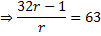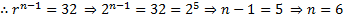# In a G.P., the sum of the first and last term is 66, the product of the second and the last but one is 128 and the sum of the terms is 126   If an increasing G.P. is considered, then the number of terms in G.P. is   a) 9 b) 8 c) 12 d) 6

## Question ID - 51125 :- In a G.P., the sum of the first and last term is 66, the product of the second and the last but one is 128 and the sum of the terms is 126   If an increasing G.P. is considered, then the number of terms in G.P. is   a) 9 b) 8 c) 12 d) 6

3537

(d)

Letbe the first term andthe common ratio of the given G.P.

Further, let there beterms in the given G.P. Then,(i)Putting this value ofin (i), we getPuttingin (1), we getPuttingin (1), we getfor an increasing G.P.,. Now,For decreasing G.P.,and. Hence, the sum of infinite terms isForterms are 2, 4, 8, 16, 32, 64. Forterms are 64, 32, 16, 8, 4, 2. Hence difference is 62

Next Question :
 Four different integers form an increasing A.P. One of these numbers is equal to the sum of the squares of the other three numbers. Then The product of all numbers is a)b) 1 c) 0 d) 2